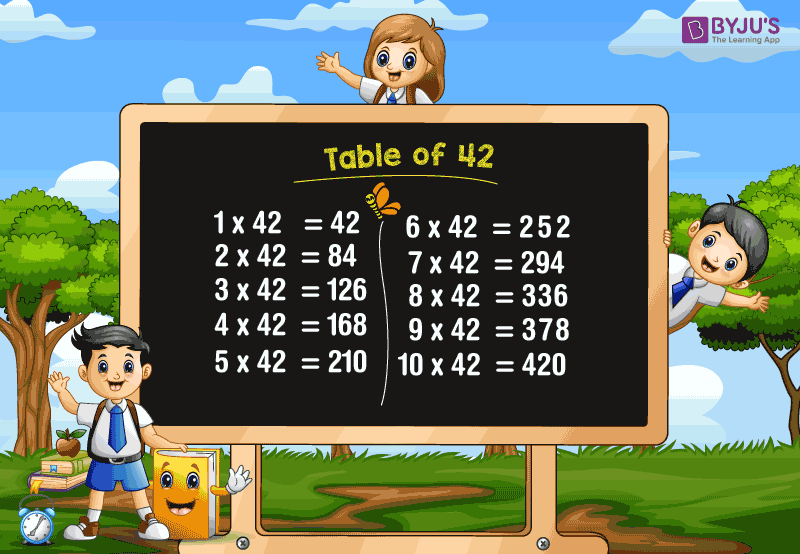# Table of 42

Table of 42 is a Maths multiplication table for the number forty-two, i.e. 42. As we know that a multiplication table is a mathematical table used to determine a multiplication operation for an algebraic method in mathematics. One can use these maths Tables from 1 to 100 while complex problems involving multiplication of numbers.

### Download Table of 42 HereWhat is the table of 42?

The table of 42 can be defined as forty-two or 42 times a number that means 42 is repeatedly added as many times as required (as per the number of times). For example, 42 times 3 means 42 has been added to itself three times.

i.e. 42 × 3 = 42 + 42 + 42 = 126

Here, we can observe the repeated addition of 42 for 3 times.

Therefore, 42 times 3 is 126, i.e., 42 × 3 = 126

Similarly, we can get the other multiples of 42. The below table shows the multiplication of forty-two with numbers from 1 to 20.

## Multiplication Table of 42

 42 × 1 = 42 42 × 2 = 84 42 × 3 = 126 42 × 4 = 168 42 × 5 = 210 42 × 6 = 252 42 × 7 = 294 42 × 8 = 336 42 × 9 = 378 42 × 10 = 420 42 × 11 = 462 42 × 12 = 504 42 × 13 = 546 42 × 14 = 588 42 × 15 = 630 42 × 16 = 672 42 × 17 = 714 42 × 18 = 756 42 × 19 = 798 42 × 20 = 840

## 42 Times Table

Read the forty-two times table from 1 to 10 given below.

• One time forty-two is forty two
• Two times forty-two is eighty four
• Three times forty-two is one hundred and twenty six
• Four times forty-two is one hundred and sixty eight
• Five times forty-two is two hundred and ten
• Six times forty-two is two hundred and fifty two
• Seven times forty-two is two hundred and ninety four
• Eight times forty-two is three hundred and thirty six
• Nine times forty-two is three hundred and seventy eight
• Ten times forty-two is four hundred and twenty

### Check More Tables

Visit www.byjus.com for more math tables and Learn math topics more engagingly and efficiently at BYJU’S – The Learning App.

## Frequently Asked Questions on Table of 42

### What is the table of 42?

The table of 42 is given as:
42 × 1 = 42, 42 × 2 = 84, 42 × 3 = 126, 42 × 4 = 168, 42 × 5 = 210, 42 × 6 = 252, 42 × 7 = 294, 42 × 8 = 336, 42 × 9 = 378, 42 × 10 = 420, etc.,

### Is 42 in the 3 times table?

Yes, we can get the number 42 in the 3 times table when we multiply 3 by 14.
That means, 3 × 14 = 42

### What is the table of 44?

The table of 44 for the numbers from 1 to 10 is given by:
44 × 1 = 44, 44 × 2 = 88, 44 × 3 = 132, 44 × 4 = 176, 44 × 5 = 220, 44 × 6 = 264, 44 × 7 = 308, 44 × 8 = 352, 44 × 9 = 396, 44 × 10 = 440.

### Is 42 in the 7 times table?

Yes, we can get the number 42 in the 7 times table when we multiply 7 by 6.
That means, 7 × 6 = 42
This can also be represented using addition as:
7 + 7 + 7 + 7 + 7 + 7 = 72

### What are the multiples of 42?

The multiples of 42 are 42, 84, 126, 168, 210, 252, 294, 336, 378, 420 and so on.